# Mean State

Period Mean (original grids) [W/m2]
Model Period Mean (intersection) [W/m2]
Model Period Mean (complement) [W/m2]
Benchmark Period Mean (intersection) [W/m2]
Benchmark Period Mean (complement) [W/m2]
Bias [W/m2]
RMSE [W/m2]
Phase Shift [months]
Bias Score 
RMSE Score 
Seasonal Cycle Score 
Spatial Distribution Score 
Interannual Variability Score 
Overall Score 
Benchmark [-] -56.1
CLM5PHSOFF [-] -65.5 -65.7 0.00 -56.5 -49.8 -9.31 16.9 1.83 0.49 0.41 0.74 0.97 0.75 0.63
CLM5PHSON [-] -65.8 -65.8 0.00 -56.5 -49.8 -9.20 16.5 1.82 0.51 0.41 0.74 0.98 0.73 0.63
Period Mean (original grids) [W/m2]
Model Period Mean (intersection) [W/m2]
Model Period Mean (complement) [W/m2]
Benchmark Period Mean (intersection) [W/m2]
Benchmark Period Mean (complement) [W/m2]
Bias [W/m2]
RMSE [W/m2]
Phase Shift [months]
Bias Score 
RMSE Score 
Seasonal Cycle Score 
Spatial Distribution Score 
Interannual Variability Score 
Overall Score 
Benchmark [-] -75.5
CLM5PHSOFF [-] -97.7 -98.3 0.00 -75.8 -54.3 -22.3 27.3 0.310 0.45 0.57 0.97 0.91 0.73 0.70
CLM5PHSON [-] -97.5 -98.2 0.00 -75.8 -54.3 -22.0 27.2 0.353 0.46 0.57 0.96 0.95 0.70 0.70
Period Mean (original grids) [W/m2]
Model Period Mean (intersection) [W/m2]
Model Period Mean (complement) [W/m2]
Benchmark Period Mean (intersection) [W/m2]
Benchmark Period Mean (complement) [W/m2]
Bias [W/m2]
RMSE [W/m2]
Phase Shift [months]
Bias Score 
RMSE Score 
Seasonal Cycle Score 
Spatial Distribution Score 
Interannual Variability Score 
Overall Score 
Benchmark [-] -76.3
CLM5PHSOFF [-] -91.1 -91.5 0.00 -77.0 -61.8 -14.5 21.7 1.05 0.48 0.52 0.87 0.99 0.78 0.69
CLM5PHSON [-] -91.0 -91.1 0.00 -77.0 -61.8 -12.1 20.7 1.11 0.53 0.50 0.86 0.99 0.76 0.69
Period Mean (original grids) [W/m2]
Model Period Mean (intersection) [W/m2]
Model Period Mean (complement) [W/m2]
Benchmark Period Mean (intersection) [W/m2]
Benchmark Period Mean (complement) [W/m2]
Bias [W/m2]
RMSE [W/m2]
Phase Shift [months]
Bias Score 
RMSE Score 
Seasonal Cycle Score 
Spatial Distribution Score 
Interannual Variability Score 
Overall Score 
Benchmark [-] -36.2
CLM5PHSOFF [-] -39.5 -41.0 0.00 -35.9 -39.8 -5.08 18.9 1.69 0.65 0.36 0.78 0.95 0.88 0.66
CLM5PHSON [-] -40.2 -40.3 0.00 -35.9 -39.8 -4.97 18.6 1.64 0.65 0.36 0.79 0.94 0.88 0.67
Period Mean (original grids) [W/m2]
Model Period Mean (intersection) [W/m2]
Model Period Mean (complement) [W/m2]
Benchmark Period Mean (intersection) [W/m2]
Benchmark Period Mean (complement) [W/m2]
Bias [W/m2]
RMSE [W/m2]
Phase Shift [months]
Bias Score 
RMSE Score 
Seasonal Cycle Score 
Spatial Distribution Score 
Interannual Variability Score 
Overall Score 
Benchmark [-] -46.4
CLM5PHSOFF [-] -46.0 -46.0 0.00 -45.1 -51.0 -1.04 12.5 2.00 0.38 0.36 0.71 0.96 0.73 0.58
CLM5PHSON [-] -47.5 -47.5 0.00 -45.1 -51.0 -1.79 12.4 1.98 0.39 0.36 0.71 0.94 0.73 0.58
Period Mean (original grids) [W/m2]
Model Period Mean (intersection) [W/m2]
Model Period Mean (complement) [W/m2]
Benchmark Period Mean (intersection) [W/m2]
Benchmark Period Mean (complement) [W/m2]
Bias [W/m2]
RMSE [W/m2]
Phase Shift [months]
Bias Score 
RMSE Score 
Seasonal Cycle Score 
Spatial Distribution Score 
Interannual Variability Score 
Overall Score 
Benchmark [-] -44.2
CLM5PHSOFF [-] -53.5 -53.5 0.00 -44.1 -53.1 -9.50 16.3 1.11 0.54 0.45 0.87 0.88 0.89 0.68
CLM5PHSON [-] -52.2 -52.2 0.00 -44.1 -53.1 -8.12 15.1 1.02 0.58 0.46 0.88 0.85 0.89 0.69
Period Mean (original grids) [W/m2]
Model Period Mean (intersection) [W/m2]
Model Period Mean (complement) [W/m2]
Benchmark Period Mean (intersection) [W/m2]
Benchmark Period Mean (complement) [W/m2]
Bias [W/m2]
RMSE [W/m2]
Phase Shift [months]
Bias Score 
RMSE Score 
Seasonal Cycle Score 
Spatial Distribution Score 
Interannual Variability Score 
Overall Score 
Benchmark [-] -66.8
CLM5PHSOFF [-] -73.2 -73.0 0.00 -67.0 -58.2 -5.92 16.9 1.16 0.64 0.51 0.85 0.98 0.82 0.72
CLM5PHSON [-] -72.9 -72.6 0.00 -67.0 -58.2 -4.65 16.5 1.10 0.67 0.51 0.86 0.99 0.83 0.73
Period Mean (original grids) [W/m2]
Model Period Mean (intersection) [W/m2]
Model Period Mean (complement) [W/m2]
Benchmark Period Mean (intersection) [W/m2]
Benchmark Period Mean (complement) [W/m2]
Bias [W/m2]
RMSE [W/m2]
Phase Shift [months]
Bias Score 
RMSE Score 
Seasonal Cycle Score 
Spatial Distribution Score 
Interannual Variability Score 
Overall Score 
Benchmark [-] -60.6
CLM5PHSOFF [-] -78.0 -78.5 0.00 -61.3 -51.8 -17.3 23.7 1.05 0.48 0.54 0.86 0.99 0.75 0.69
CLM5PHSON [-] -79.0 -79.2 0.00 -61.3 -51.8 -15.1 22.5 1.07 0.52 0.52 0.86 0.99 0.74 0.69
Period Mean (original grids) [W/m2]
Model Period Mean (intersection) [W/m2]
Model Period Mean (complement) [W/m2]
Benchmark Period Mean (intersection) [W/m2]
Benchmark Period Mean (complement) [W/m2]
Bias [W/m2]
RMSE [W/m2]
Phase Shift [months]
Bias Score 
RMSE Score 
Seasonal Cycle Score 
Spatial Distribution Score 
Interannual Variability Score 
Overall Score 
Benchmark [-] -32.3
CLM5PHSOFF [-] -40.8 -40.9 0.00 -32.2 -34.9 -8.71 18.9 2.12 0.56 0.36 0.71 0.92 0.90 0.64
CLM5PHSON [-] -40.5 -40.5 0.00 -32.2 -34.9 -8.31 18.5 2.10 0.57 0.37 0.72 0.94 0.90 0.64
Period Mean (original grids) [W/m2]
Model Period Mean (intersection) [W/m2]
Model Period Mean (complement) [W/m2]
Benchmark Period Mean (intersection) [W/m2]
Benchmark Period Mean (complement) [W/m2]
Bias [W/m2]
RMSE [W/m2]
Phase Shift [months]
Bias Score 
RMSE Score 
Seasonal Cycle Score 
Spatial Distribution Score 
Interannual Variability Score 
Overall Score 
Benchmark [-] -88.6
CLM5PHSOFF [-] -95.8 -95.9 0.00 -90.4 -66.1 -5.55 15.0 0.799 0.66 0.53 0.89 0.99 0.85 0.74
CLM5PHSON [-] -98.3 -98.4 0.00 -90.4 -66.1 -4.59 15.0 0.857 0.67 0.53 0.89 0.99 0.84 0.74
Period Mean (original grids) [W/m2]
Model Period Mean (intersection) [W/m2]
Model Period Mean (complement) [W/m2]
Benchmark Period Mean (intersection) [W/m2]
Benchmark Period Mean (complement) [W/m2]
Bias [W/m2]
RMSE [W/m2]
Phase Shift [months]
Bias Score 
RMSE Score 
Seasonal Cycle Score 
Spatial Distribution Score 
Interannual Variability Score 
Overall Score 
Benchmark [-] -54.5
CLM5PHSOFF [-] -60.4 -60.3 0.00 -54.0 -59.9 -6.30 14.2 1.51 0.62 0.46 0.78 0.99 0.88 0.70
CLM5PHSON [-] -58.1 -58.1 0.00 -54.0 -59.9 -4.66 13.9 1.59 0.66 0.45 0.77 0.98 0.88 0.70
Period Mean (original grids) [W/m2]
Model Period Mean (intersection) [W/m2]
Model Period Mean (complement) [W/m2]
Benchmark Period Mean (intersection) [W/m2]
Benchmark Period Mean (complement) [W/m2]
Bias [W/m2]
RMSE [W/m2]
Phase Shift [months]
Bias Score 
RMSE Score 
Seasonal Cycle Score 
Spatial Distribution Score 
Interannual Variability Score 
Overall Score 
Benchmark [-] -53.4
CLM5PHSOFF [-] -74.8 -71.3 0.00 -61.2 -49.6 -10.1 19.0 1.31 0.54 0.47 0.82 0.98 0.78 0.68
CLM5PHSON [-] -71.7 -71.7 0.00 -61.2 -49.6 -9.06 18.5 1.31 0.56 0.47 0.82 0.98 0.77 0.68
Period Mean (original grids) [W/m2]
Model Period Mean (intersection) [W/m2]
Model Period Mean (complement) [W/m2]
Benchmark Period Mean (intersection) [W/m2]
Benchmark Period Mean (complement) [W/m2]
Bias [W/m2]
RMSE [W/m2]
Phase Shift [months]
Bias Score 
RMSE Score 
Seasonal Cycle Score 
Spatial Distribution Score 
Interannual Variability Score 
Overall Score 
Benchmark [-] -100.
CLM5PHSOFF [-] -108. -108. 0.00 -101. -73.0 -6.63 16.9 0.849 0.63 0.51 0.88 0.97 0.80 0.72
CLM5PHSON [-] -108. -108. 0.00 -101. -73.0 -6.02 16.9 0.860 0.63 0.51 0.88 0.98 0.80 0.72
Period Mean (original grids) [W/m2]
Model Period Mean (intersection) [W/m2]
Model Period Mean (complement) [W/m2]
Benchmark Period Mean (intersection) [W/m2]
Benchmark Period Mean (complement) [W/m2]
Bias [W/m2]
RMSE [W/m2]
Phase Shift [months]
Bias Score 
RMSE Score 
Seasonal Cycle Score 
Spatial Distribution Score 
Interannual Variability Score 
Overall Score 
Benchmark [-] -33.5
CLM5PHSOFF [-] -44.0 -44.0 0.00 -33.5 -34.8 -10.6 19.3 2.12 0.55 0.39 0.68 0.99 0.84 0.64
CLM5PHSON [-] -43.3 -43.3 0.00 -33.5 -34.8 -10.2 19.1 2.13 0.56 0.39 0.68 0.99 0.84 0.64
Period Mean (original grids) [W/m2]
Model Period Mean (intersection) [W/m2]
Model Period Mean (complement) [W/m2]
Benchmark Period Mean (intersection) [W/m2]
Benchmark Period Mean (complement) [W/m2]
Bias [W/m2]
RMSE [W/m2]
Phase Shift [months]
Bias Score 
RMSE Score 
Seasonal Cycle Score 
Spatial Distribution Score 
Interannual Variability Score 
Overall Score 
Benchmark [-] -66.0
CLM5PHSOFF [-] -82.5 -82.7 0.00 -67.8 -57.2 -14.9 22.1 2.10 0.40 0.44 0.65 0.96 0.81 0.62
CLM5PHSON [-] -84.7 -84.6 0.00 -67.8 -57.2 -13.3 20.9 2.06 0.44 0.44 0.65 0.97 0.81 0.62
Period Mean (original grids) [W/m2]
Model Period Mean (intersection) [W/m2]
Model Period Mean (complement) [W/m2]
Benchmark Period Mean (intersection) [W/m2]
Benchmark Period Mean (complement) [W/m2]
Bias [W/m2]
RMSE [W/m2]
Phase Shift [months]
Bias Score 
RMSE Score 
Seasonal Cycle Score 
Spatial Distribution Score 
Interannual Variability Score 
Overall Score 
Benchmark [-] -51.6
CLM5PHSOFF [-] -65.2 -65.2 0.00 -51.4 -56.8 -13.8 17.8 1.91 0.34 0.42 0.68 0.84 0.90 0.60
CLM5PHSON [-] -62.9 -62.7 0.00 -51.4 -56.8 -11.3 15.7 1.88 0.42 0.42 0.70 0.82 0.90 0.61
Period Mean (original grids) [W/m2]
Model Period Mean (intersection) [W/m2]
Model Period Mean (complement) [W/m2]
Benchmark Period Mean (intersection) [W/m2]
Benchmark Period Mean (complement) [W/m2]
Bias [W/m2]
RMSE [W/m2]
Phase Shift [months]
Bias Score 
RMSE Score 
Seasonal Cycle Score 
Spatial Distribution Score 
Interannual Variability Score 
Overall Score 
Benchmark [-] -46.1
CLM5PHSOFF [-] -54.6 -54.6 0.00 -46.1 -44.3 -8.44 14.4 0.785 0.60 0.50 0.91 0.93 0.86 0.72
CLM5PHSON [-] -53.2 -53.2 0.00 -46.1 -44.3 -6.84 13.5 0.817 0.65 0.50 0.91 0.89 0.86 0.72
Period Mean (original grids) [W/m2]
Model Period Mean (intersection) [W/m2]
Model Period Mean (complement) [W/m2]
Benchmark Period Mean (intersection) [W/m2]
Benchmark Period Mean (complement) [W/m2]
Bias [W/m2]
RMSE [W/m2]
Phase Shift [months]
Bias Score 
RMSE Score 
Seasonal Cycle Score 
Spatial Distribution Score 
Interannual Variability Score 
Overall Score 
Benchmark [-] -78.8
CLM5PHSOFF [-] -89.3 -89.1 0.00 -79.2 -53.1 -10.0 17.8 1.20 0.62 0.55 0.83 0.99 0.86 0.73
CLM5PHSON [-] -88.1 -87.9 0.00 -79.2 -53.1 -8.35 17.1 1.20 0.65 0.54 0.83 0.99 0.87 0.74
Period Mean (original grids) [W/m2]
Model Period Mean (intersection) [W/m2]
Model Period Mean (complement) [W/m2]
Benchmark Period Mean (intersection) [W/m2]
Benchmark Period Mean (complement) [W/m2]
Bias [W/m2]
RMSE [W/m2]
Phase Shift [months]
Bias Score 
RMSE Score 
Seasonal Cycle Score 
Spatial Distribution Score 
Interannual Variability Score 
Overall Score 
Benchmark [-] -39.8
CLM5PHSOFF [-] -52.3 -52.1 0.00 -39.6 -48.2 -12.6 17.6 1.67 0.33 0.42 0.76 0.90 0.70 0.59
CLM5PHSON [-] -51.8 -51.6 0.00 -39.6 -48.2 -12.1 17.0 1.70 0.33 0.43 0.76 0.96 0.68 0.60
Period Mean (original grids) [W/m2]
Model Period Mean (intersection) [W/m2]
Model Period Mean (complement) [W/m2]
Benchmark Period Mean (intersection) [W/m2]
Benchmark Period Mean (complement) [W/m2]
Bias [W/m2]
RMSE [W/m2]
Phase Shift [months]
Bias Score 
RMSE Score 
Seasonal Cycle Score 
Spatial Distribution Score 
Interannual Variability Score 
Overall Score 
Benchmark [-] -63.6
CLM5PHSOFF [-] -73.8 -74.2 0.00 -63.7 -59.9 -10.5 17.2 1.25 0.56 0.49 0.84 0.98 0.80 0.69
CLM5PHSON [-] -72.4 -72.8 0.00 -63.7 -59.9 -8.42 16.1 1.27 0.62 0.48 0.83 0.98 0.77 0.70
Period Mean (original grids) [W/m2]
Model Period Mean (intersection) [W/m2]
Model Period Mean (complement) [W/m2]
Benchmark Period Mean (intersection) [W/m2]
Benchmark Period Mean (complement) [W/m2]
Bias [W/m2]
RMSE [W/m2]
Phase Shift [months]
Bias Score 
RMSE Score 
Seasonal Cycle Score 
Spatial Distribution Score 
Interannual Variability Score 
Overall Score 
Benchmark [-] -80.2
CLM5PHSOFF [-] -84.9 -84.6 0.00 -83.6 -50.6 -0.767 21.7 1.22 0.54 0.46 0.84 0.93 0.77 0.67
CLM5PHSON [-] -85.8 -85.5 0.00 -83.6 -50.6 1.06 21.5 1.21 0.56 0.46 0.84 0.92 0.78 0.67

# Temporally integrated period mean

BENCHMARK MEAN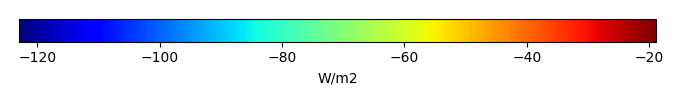MODEL MEANBIAS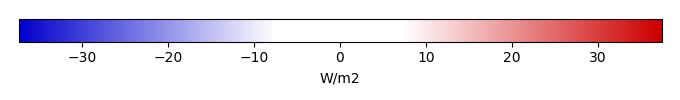BIAS SCORERMSE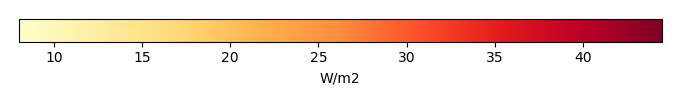RMSE SCOREBENCHMARK INTERANNUAL VARIABILITY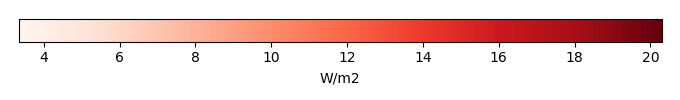MODEL INTERANNUAL VARIABILITYINTERANNUAL VARIABILITY SCOREBENCHMARK MAX MONTHMODEL MAX MONTHDIFFERENCE IN MAX MONTH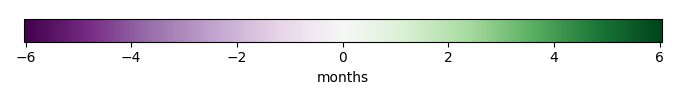SEASONAL CYCLE SCORESPATIAL TAYLOR DIAGRAMMODEL COLORS# Spatially integrated regional mean

MODEL COLORSREGIONAL MEANANNUAL CYCLEMONTHLY ANOMALYANNUAL CYCLE# All Models

BenchmarkCLM5PHSOFFCLM5PHSON# Data Information

creation_date: Thu May 8 23:08:21 PDT 2014

source_file: This product is generated from monthly 1 degree CERES EBAF Radiation observations